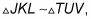##### Geometry For DummiesIf you get a problem with a diagram of similar polygons that aren’t lined up in the same orientation, consider redrawing one of them so that they’re both aligned in the same way. This may make the problem easier to solve.

Here are a few things you can do to help you see how the vertices of similar polygons match up when the polygons are positioned differently:

• You can often tell how the vertices correspond just by looking at the polygons, which is actually a pretty good way of seeing whether one polygon has been flipped over or spun around.

• If the similarity is given to you and written out likeyou know that the first letters, J and T, correspond, K and U correspond, and L and V correspond. The order of the letters also tells you that segment KL corresponds to segment UV, and so on.

• If you know the measures of the angles or which angles are congruent to which, that information tells you how the vertices correspond because corresponding angles in similar polygons are congruent.

• If you’re given (or you figure out) which sides are proportional, that info tells you how the sides would stack up, and from that you can see how the vertices correspond.